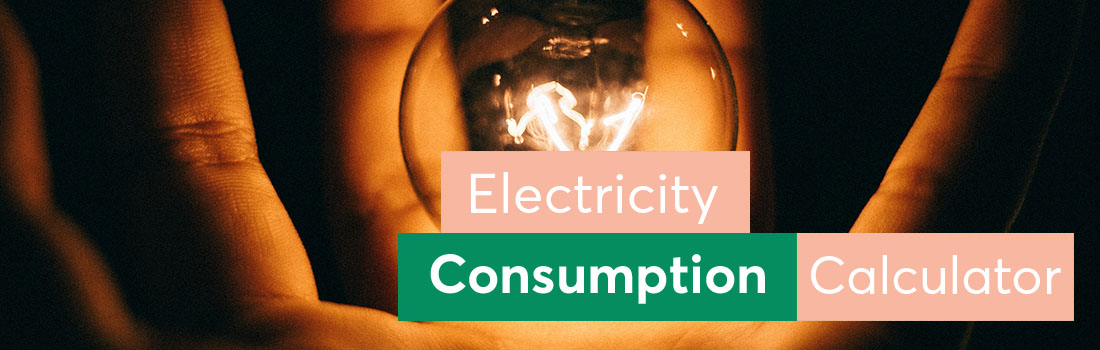# Electricity Consumption Calculator - Online Tool

A watt consumption calculator helps you determine how much it costs to run your electrical devices. You simply use the wattage of each appliance that you have installed and the calculator will tell you how much you will have to spend on electricity for the device per year. You can find quite a few energy consumption calculator tools online.

## How does an electricity usage calculator work?

These are the steps to follow:

### ~ Step 1 ~

In the power consumption calculator, enter the wattage consumption of your appliance and how long it is used each day.

### ~ Step 2 ~

Enter the 1Kwh energy price.

### ~ Step 3 ~

The calculator will compute your annual electricity use.## How do you figure out how much electricity you use?

To calculate the energy usage (kWh) with a power usage calculator, multiply the wattage of the device (W or kW) by the total time or number of hours used. For example, if you watch a 300W TV for four hours each day, you will consume 1200Wh or 1.2kWh per day. The usage cost for one month is:

1.2kWh x 30 days x 0.20 \$/kWh) = \$7.20

Watts are electrical units of measurement. A Watt is an electrical power unit equivalent to one ampere at one volt of pressure. In the metric system, one watt equals one joule for each second of energy that flows.

## What exactly is a Kilowatt Hour or KWh?

It is one of the fundamental units used to measure energy usage. To give you an idea, this amount corresponds to how much a machine works with a one-kilowatt output for one hour. In practice, you will also come across the unit megawatt-hour, which is equal to 1,000 kilowatt-hours.

## Calculating Your Power Usage

An application's operational time might help you calculate your electricity expenses. The more your home's electronic devices operate during the day, the more electricity they consume, resulting in increased power consumption. Remember to enter the time of operation of your appliance into the power usage calculator that you use.

## How to Know the Average Electricity Usage in an Average Home?

If you get an energy bill every month, you probably already have an idea about how much you are paying by way of your energy expenses. However, do you know how your household's energy use is, as compared to the national average? You might be astonished to learn how much more of electricity your home uses than others. The average annual power usage for a household utility user in the United States is 11 kilowatt-hours (kWh).

Its 29 kWh every day divided by 365.You can use an energy usage calculator to determine the expenses and calculate kWh usage.

## How can you lower your power consumption?

These are a few steps to follow:

### 1. Turn off your computer

Computers are among the most energy-intensive devices in office buildings. Turn off your monitor at night and remove the screensaver. Computers today can be switched on and off over 40,000 times.

### 2. Select appropriate lighting fixtures

LED bulbs are among the most energy-saving illuminants. LED lamps consume 75% less energy than incandescent bulbs.

### 3. Remove vampire power by unplugging unused electronics

Even when turned off, devices such as scanners, microwaves, televisions and printers utilize stand-by power. Even when plugged in, a few chargers go on drawing modest amount of energy.

### 4. Go for appliances that are efficient

You should purchase energy-efficient appliances. Look for an Energy Star rating, which is a federal assurance that the product will consume less energy.

### 5. Reduce water consumption

Only wash full clothing loads in the washing machine with cold water. Instead of using a dishwasher, use hands to wash dishes; sometimes at least.